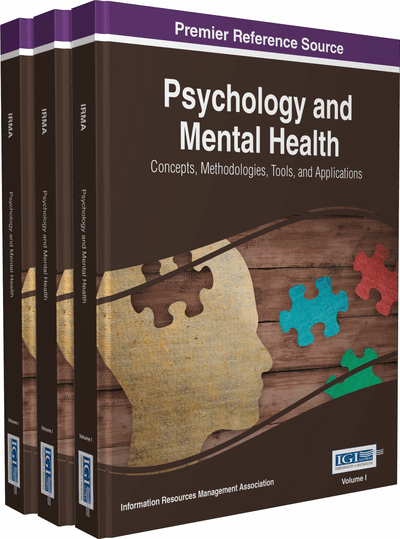# Neural Networks Predictive Controller Using an Adaptive Control Rate

Ahmed Mnasser (Tunis El Manar University, Tunisia), Faouzi Bouani (Tunis El Manar University, Tunisia) and Mekki Ksouri (Tunis El Manar University, Tunisia)
DOI: 10.4018/978-1-5225-0159-6.ch026
Available
\$37.50
No Current Special Offers

## Abstract

A model predictive control design for nonlinear systems based on artificial neural networks is discussed. The Feedforward neural networks are used to describe the unknown nonlinear dynamics of the real system. The backpropagation algorithm is used, offline, to train the neural networks model. The optimal control actions are computed by solving a nonconvex optimization problem with the gradient method. In gradient method, the steepest descent is a sensible factor for convergence. Then, an adaptive variable control rate based on Lyapunov function candidate and asymptotic convergence of the predictive controller are proposed. The stability of the closed loop system based on the neural model is proved. In order to demonstrate the robustness of the proposed predictive controller under set-point and load disturbance, a simulation example is considered. A comparison of the control performance achieved with a Levenberg-Marquardt method is also provided to illustrate the effectiveness of the proposed controller.
Chapter Preview
Top

## 1. Introduction

Model Predictive Control (MPC) has been widely implemented in the industrial processes (Holkar & Waghmare, 2010). It belongs to a general class of predictive control. Numerous implementation algorithms have been addressed to this topic which are published in literature (Abbaszadeh, 2011; Rawling, 2000). The control problem involves an objective function subject to constraints which are generally associated to the inputs and outputs of the system (Garcia et al., 1989). The MPC is based on the use of a mathematical model to predict the future outputs of the plant over prediction horizon. Therefore, the success of the MPC depends on the precision of the model of the system to be controlled. The MPC technique based on linear model is not very efficient in the control of nonlinear system (Lawrynczuk, 2011). However, the increasing complex and highly nonlinear dynamical systems have limited the use of linear model based controllers. Consequently, a very large variety of control techniques have been presented based on a nonlinear model of the system (Li & Kashiwagi, 2005). The MPC approach is founded on the computation of output predictions for an arbitrary prediction horizon based on system model. The most often used models in real applications based on the MPC approach are state-space and input-output models. For the first model, the computation of the output predictions of the system is rather obvious. For the second one, there exist several techniques how to calculate the output prediction equations. However, the MPC approach depends on the model used to characterize the system’s behavior and on the objective function used to compute the control law. Numerous approaches have been used to approximate the unknown nonlinear behavior of the system, such as polynomial models and neural networks models.

The synthesis of the predictive controller based on linear model gives a convex optimization problem (Clarke et al., 1987; Kheriji et al., 2011). Then, local optimization methods provide the global optimum of the optimization problem. In cases of MPC based on a nonlinear model, including Wiener model, Volterra model, and neural model, the objective function to be optimized is nonconvex. Solving this class of problem by local optimization methods provides local solutions due to the non convexity of the objective function. Several authors have used some methods to reach the global optimum of the nonconvex problem (Bouzouita et al., 2008; Mnasser & Bouani, 2014).

Artificial neural networks are a powerful tool for modeling system and have been widely used to solve many problems related to control system for industrial processes which are characterized by complexity and highly nonlinearities (Azar, 2012; Mnasser & Bouani, 2014; Li & Cheah, 2014; Tong et al., 2011; Narendra, 1996; Pan & Wang, 2012). Since the introduction of the McCulloch and Pitts neural networks model in 1940s, several others neural network models have been developed in research (Fu, 1995; Azar, 2013; Azar & El-Said, 2013). Neural network models for solving optimization problem and their industrial applications have been widely investigated in the past three decades (El-Said, 2013; Taher & Abdeljawed, 2013). They are the most popular in modeling of the complex systems, including the Feedforward Neural Networks (FNNs). The FNNs show their ability to approximate any unknown nonlinear function since the establishment of the universal approximator theory (Hornic & Stinchombe, 1989). The successful application of the MPC based on a neural networks model for nonlinear systems was appeared in an accurate nonlinear model and an efficient control algorithm (Mnasser et al., 2010; Narendra, 1996; Pan & Wang, 2012).

## Complete Chapter List

Search this Book:
Reset